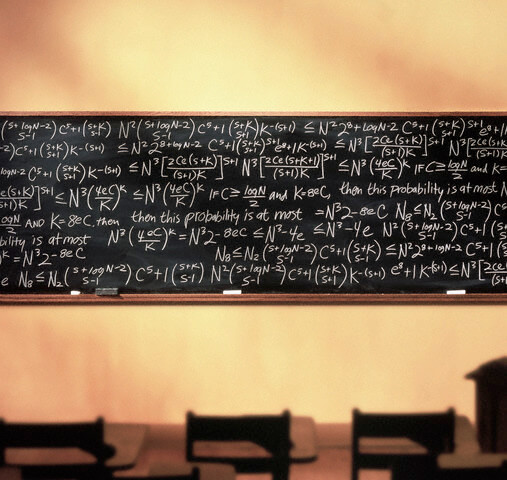The roulette problem.
(Un)predictability, Probability, and Stupidity. Or Mathematics and Timing in Roulette systems. Or going beyond the Kelly Criterion.1. The Predictability factor. The unpredictable nature of roulette is much more complex than “the outcome of the next spin”. Since we wot bet all our money in the next spin, we don’t care specifically for it. We can stand to lose a few spins if we will win more in time. Probability theory offers us some very valuable knowledge, that helps us predict the general trend of the outcomes. However, this knowledge alone cannot guarantee profits. For example: if we (somehow) knew that in the next 100 spins there will be 45 Blacks (rest 55 spins are unknown consisting of zeros and red), this knowledge is not enough to make a profit. Especially if we have limited capital.

2. The timing factor. So even if we can predict the general image of future outcomes, this is not enough to make a profit. The problem is that we still do not know the exact “timing” of these outcomes. Will these 45 Blacks come early or late in these 100 spins? Will they come in long or short series?

3. Lack of mathematical research of roulette problems. Here is where a really good mathematician could offer some much needed help. And it a real waste of their knowledge and time for math boyz to keep repeating all the nonsense about the house edge etc. But you know what? It’s much easier to repeat aphorisms than to try to solve difficult mathematical problems. What a shame.

The Problem

You have a bankroll of 100 units.
You know for sure that in the next 100 spins, 45 will be BLACK.
You don’t know the outcome of the rest 55 spins, could be reds or zeros or even blacks.
Which is, mathematically, the best way to bet, in order to make the surest and maximum profit?

I’m not holding my breath for an answer. Serious mathematicians able and willing to solve tis type of problems are 1 in a million. It is a very difficult problem indeed. Because the is a multitude of possible 100 spin series. One has to find an equation that can be valid for (adapt to) any possible outcomes, while taking into account our limited capital. Yes, this little roulette problem is the kind of problem that only mathematicians of the Like John Kelly, Shannon, Thorp or Bernoulli could solve. Who were bold enough to try and give answers to similar problems. Like the Kelly criterion and the St. Petersburg paradox. This type of questions are too deep waters for the self proclaimed probability, mathematics and gambling experts. You won’t get a valid answer – he’ll probably tell you, you can’t make a profit since you only know the result of 45 spins. Math boys won’t touch such problems. Let them hide behind the house edge.

People are asking me about the practical value of this problem. “You can never be sure there will be 45 blacks in 100 spins, in the first place. So why ask this question anyway?” The answer is that there are instances in which we can indeed predict the future outcomes i general terms. For example, as already mentioned in this extremely interesting thread in roulette 30 forum it is impossible in 200 spins to have less than 65 Blacks. So if in the first 100 spins we had, say, less than 25 Blacks is right to assume than in the next 100 spins we will have at least 45 Blacks. The truth is that someone familiar with roulette probabilities and expectations will can make often predictions like these with a success rate that could provide profits. IF only we knew the best way to bet these spins, which is what the above problem is all about.